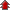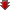Home Board Paper Solutions Text Book Solutions Articles NCERT Exemplar Solutions

# Electrochemistry### Exercise 1

•  Q1 How would you determine the standard electrode potential of the system Mg2+ | Mg? Q2 Can you store copper sulphate solutions in a zinc pot? Q3 Consult the table of standard electrode potentials and suggest three substances that can oxidise ferrous ions under suitable conditions.">Consult the table of standard electrode potentials and suggest three substances that can oxidise ferrous ions under suitable conditions. Q4 Calculate the potential of hydrogen electrode in contact with a solution whose pH is 10.">Calculate the potential of hydrogen electrode in contact with a solution whose pH is 10. Q5 Calculate the emf of the cell in which the following reaction takes place: Ni(s) + 2Ag+ (0.002 M) → Ni2+ (0.160 M) + 2Ag(s) Given that Eøcell = 1.05 V Q6 has Eøcell = 0.236 V at 298 K. Calculate the standard Gibbs energy and the equilibrium constant of the cell reaction.">The cell in which the following reactions occurs:has Eøcell = 0.236 V at 298 K. Calculate the standard Gibbs energy and the equilibrium constant of the cell reaction. Q7 Why does the conductivity of a solution decrease with dilution? Q9 The molar conductivity of 0.025 mol L-1 methanoic acid is 46.1 S cm2 mol-1. Calculate its degree of dissociation and dissociation constant. Given λ0(H+) = 349.6 S cm2 mol-1 and  λ0(HCOO-) = 54.6 S cm2 mol Q10 If a current of 0.5 ampere flows through a metallic wire for 2 hours, then how many electrons would flow through the wire?">If a current of 0.5 ampere flows through a metallic wire for 2 hours, then how many electrons would flow through the wire? Q11 Suggest a list of metals that are extracted electrolytically.">Suggest a list of metals that are extracted electrolytically. Q12 Consider the reaction: Cr2 O72- + 14H+ + 6e- → Cr3+ + 8H2O  What is the quantity of electricity in coulombs needed to reduce 1 mol of Cr2 O72-? Q13 Write the chemistry of recharging the lead storage battery, highlighting all the materials that are involved during recharging.">Write the chemistry of recharging the lead storage battery, highlighting all the materials that are involved during recharging. Q14 Suggest two materials other than hydrogen that can be used as fuels in fuel cells. Q15 Explain how rusting of iron is envisaged as setting up of an electrochemical cell.### Exercise 2

•  Q1 Arrange the following metals in the order in which they displace each other from the solution of their salts. Al, Cu, Fe, Mg and Zn Q2 Given the standard electrode potentials, K+/ K = - 2.93V,  Ag+/ Ag = 0.80V, Hg2+ / Hg = 0.79V Mg2+ / Mg = - 2.37 V, Cr3+ / Cr = - 0.74V Arrange these metals in their increasing order of reducing power. Q3 Depict the galvanic cell in which the reaction Zn(s) + 2Ag+(aq) → Zn2+(aq) + 2Ag(s) takes place. Further show: (i) Which of the electrode is negatively charged? (ii) The carriers of the current in the cell. (iii) Individual reaction at each electrode. Q4 Calculate the standard cell potentials of galvanic cells in which the following reactions take place: (i) 2Cr(s) + 3Cd2+(aq) → 2Cr3+(aq) + 3Cd (ii) Fe2+(aq) + Ag+(aq) → Fe3+(aq) + Ag(s) Calculate the ΔrGø¸ and equilibrium constant of the reactions. Q5 Write the Nernst equation and emf of the following cells at 298 K: (i) Mg(s) | Mg2+(0.001M) || Cu2+(0.0001 M) | Cu(s) (ii) Fe(s) | Fe2+(0.001M) || H+(1M)|H2(g)(1bar) | Pt(s) (iii) Sn(s) | Sn2+(0.050 M) || H+(0.020 M) | H2(g) (1 bar) | Pt(s) (iv) Pt(s) | Br2(l) | Br-(0.010 M) || H+(0.030 M) | H2(g) (1 bar) | Pt(s). Q6 In the button cells widely used in watches and other devices the following reaction takes place: Zn(s) + Ag2O(s) + H2O(l)  → Zn2+(aq) + 2Ag(s) + 2OH - (aq) Determine and for the reaction. Q7 Define conductivity and molar conductivity for the solution of an electrolyte. Discuss their variation with concentration.">Define conductivity and molar conductivity for the solution of an electrolyte. Discuss their variation with concentration. Q8 The conductivity of 0.20 M solution of KCl at 298 K is 0.0248 Scm-1. Calculate its molar conductivity. Q9 The resistance of a conductivity cell containing 0.001M KCl solution at 298 K is 1500 Ω. What is the cell constant if conductivity of 0.001M KCl solution at 298 K is 0.146 x 10-3 S cm-1.">The resistance of a conductivity cell containing 0.001M KCl solution at 298 K is 1500 Ω. What is the cell constant if conductivity of 0.001M KCl solution at 298 K is 0.146 x 10-3 S cm-1. Q11 Conductivity of 0.00241 M acetic acid is 7.896 × 10 - 5 S cm - 1. Calculate its molar conductivity and if Amº for acetic acid is 390.5 S cm2 mol - 1, what is its dissociation constant?  ">Conductivity of 0.00241 M acetic acid is 7.896 × 10 - 5 S cm - 1. Calculate its molar conductivity and if Amº for acetic acid is 390.5 S cm2 mol - 1, what is its dissociation constant? Q12 How much charge is required for the following reductions: (i) 1 mol of Al3+ to Al. (ii) 1 mol of Cu2+ to Cu. (iii) 1 mol of MnO4- to Mn2+. Q13 How much electricity in terms of Faraday is required to produce (i) 20.0 g of Ca from molten CaCl2. (ii) 40.0 g of Al from molten Al2O3. Q14 How much electricity is required in coulomb for the oxidation of (i) 1 mol of H2O to O2. (ii) 1 mol of FeO to Fe2O3. Q15 A solution of Ni(NO3)2 is electrolysed between platinum electrodes using a current of 5 amperes for 20 minutes. What mass of Ni is deposited at the cathode?  ">A solution of Ni(NO3)2 is electrolysed between platinum electrodes using a current of 5 amperes for 20 minutes. What mass of Ni is deposited at the cathode? Q16 Three electrolytic cells A,B,C containing solutions of ZnSO4, AgNO3 and CuSO4, respectively are connected in series. A steady current of 1.5 amperes was passed through them until 1.45 g of silver deposited at the cathode of cell B. How long did the current flow? What mass of copper and zinc were deposited?">Three electrolytic cells A,B,C containing solutions of ZnSO4, AgNO3 and CuSO4, respectively are connected in series. A steady current of 1.5 amperes was passed through them until 1.45 g of silver deposited at the cathode of cell B. How long did the current flow? What mass of copper and zinc were deposited? Q17 Using the standard electrode potentials given in Table 3.1, predict if the reaction between the following is feasible: (i) Fe3+(aq) and I-(aq) (ii) Ag+ (aq) and Cu(s) (iii) Fe3+ (aq) and Br- (aq) (iv) Ag(s) and Fe3+ (aq) (v) Br2 (aq) and Fe2+ (aq). Q18 Predict the products of electrolysis in each of the following: (i) An aqueous solution of AgNO3 with silver electrodes. (ii) An aqueous solution of AgNO3with platinum electrodes. (iii) A dilute solution of H2SO4with platinum electrodes. (iv) An aqueous solution of CuCl2 with platinum electrodes.

## Recently Viewed Questions of Class 12th chemistry

• NCERT Chapter